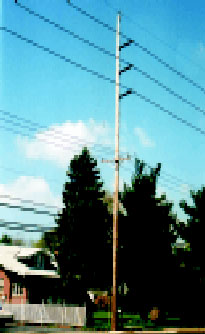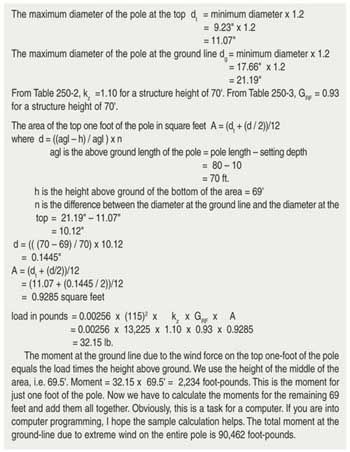In the last IAEI News (March/April 2002), I shared with you the details of the new extreme wind loading requirements of the 2002 National Electrical Safety Code (NESC). For structures sixty feet tall and shorter, the extreme wind loading only applies to the structure. For structures taller than sixty feet, the extreme wind loading applies to the structure and all the supported facilities. To understand the impact of the 2002 revision, lets crank through an example calculation.Photo 1

Consider the following example shown in photo 1 [beginning of article]:

Structures:80-foot southern yellow pine poles set 10 feet in the ground

All spans are 200′.

Supported Facilities:

1-7 No. 5 AW static wire at 70′, conductor diameter 0.546″

1-795-30/19 ACSR 138 kV phase wire at 60′, conductor diameter 1.140″

1-795-30/19 ACSR 138 kV phase wire at 54′, conductor diameter 1.140″

1-795-30/19 ACSR 138 kV phase wire at 48′, conductor diameter 1.140″

3-477 AAC 12 kV phase wires at 38′, conductor diameter 0.793″

1-1/0 AAAC neutral wire at 28′, conductor diameter 0.398″

1-1.5″ diameter communications cable at 25′

1-1.5″ diameter communications cable at 24′

1-0.5″ diameter communications cable at 23′

1-3″ diameter communications cable at 21′

Question

What is the minimum pole class (diameter) necessary to withstand the 2002 NESC extreme wind loading?

To determine the minimum pole class, we will calculate the total moment on the pole at the ground-line by multiplying the wind load on the pole and all supported facilities by the height of attachment of those facilities. The calculation will be done for the worst-case condition, when the wind is blowing perpendicular to the line. For this example, assume the line will be constructed on the East coast in an area where the Basic Wind speed per Figure 250-2(b), page 167, is 115 miles per hour. The first step is to calculate the wind load on the pole.Table 1

Because the pole diameter changes over its length, we will look at the wind load on each one-foot segment of the pole, starting at the top. To do this calculation, we must assume a particular pole class, calculate the moment, and then check to see if the class we assumed is adequate. The minimum dimensions of wood poles are given in ANSI Standard O5.1 Specifications and Dimensions for Wood Poles. Minimum pole circumferences are given by wood type at the top of the pole and six feet from the butt. Dimensions at other locations can be determined by interpolation. For an 80-foot class H1 pole, the minimum diameter at the top is 9.23 inches and the minimum diameter at the ground line, i.e., 10 feet from the butt, is 17.66 inches. For wind load calculations, we have to use maximum dimensions so we multiply the minimums by 1.2 as suggested by section 6.2.2, page 7, of the ANSI O5.1 standard. From Rule 250C, page 161, for cylindrical structures and attachments:

load in pounds = 0.00256 x (wind speed)2x kzx GRFx Area

The velocity pressure exposure coefficients kzfor structures are given in Table 250-2, page 163, as a function of the height of the structure above ground. The gust response factors GRFfor structures are given in Table 250-3 as a function of height of the structure above ground. The velocity pressure exposure coefficients kz for conductors are given in Table 250-2 as a function of the height of attachment of the conductor on the structure. The gust response factors GRFfor conductors are given in Table 250-3 as a function of height of attachment on the structure and conductor span length (see table 1).

To calculate the wind load on the conductors, we use the same equation for load in pounds except kzand GRFare different for conductors and the Area A is the cross-sectional area of the conductor for half the span in each direction. The moment for each conductor is the load multiplied times the height of attachment for that conductor. For the 7 No. 5 AW static wire, the height of attachment is 70′. From Table 250-2, kzis 1.20 for a conductor 70 feet above ground. From Table 250-3, GRF is 0.86 for a conductor 70′ above ground and spans shorter than 250′. Since the spans are all 200 feet, the conductor area in square feet is the conductor length in feet multiplied by the conductor diameter in feet. The conductor diameter in feet is 0.546″ / 12 = 0.0455. The conductor area A is then 200 x 0.0455 = 9.1 square feet.

= 0.00256 x (115)2x kzx GRFx A

= 0.00256 x 13,225 x 1.20 x 0.86 x 9.1

= 317.9

The moment on the pole at the ground-line due to the extreme wind load on the static wire is

317.9 x 70′ = 22,253 foot-pounds

We then calculate the moments due to the other conductors and add them all. At 38′, we have three 477 AAC conductors. Calculate the moment for one conductor and then multiply the answer by three. The total moment due to extreme wind on all the conductors is 221,764 foot-pounds compared to 90,462 for the structure. The large communications cables contribute 77,661 foot-pounds even though they are close to the ground. The total moment is 312,226 foot-pounds.

Safety Factors

Rule 260B1, page 175, states that “Structures shall be designed to withstand the appropriate loads multiplied by the overload factors in Section 25 without exceeding their strength multiplied by the strength factors of Section 26.” The safety factor is the overload factor divided by the strength factor. Table 253-2, page 174, gives us the overload factor of 1.33 for use with 250C loads (extreme wind loads). The corresponding strength factor of 1.0 is given in Table 261-1B, page 182. The safety factor is 1.33 / 1.0 = 1.33. The calculated moments must be multiplied by the safety before comparison to the pole strength.

Total moment with safety factor

= 312,226 x 1.33 = 415,260 foot-pounds.

Pole Strength

The pole strength or ultimate moment at the ground-line for a southern yellow pine pole is 2.111 multiplied by the cube of the pole circumference in inches.

The minimum circumference at the ground-line

= ( total moment / 2.111)1/3

= (415,260 / 2.111)1/3

= 58.16″

The minimum diameter

= circumference / 3.1416

= 58.16 / 3.1416

= 18.51″

The minimum diameter for an 80-foot H1 class pole at ground line is 18.11″. It looks like we need an H2 pole (19.05″). Since we assumed an H1 pole for the wind loading on the pole calculation, we will have to recalculate for a H2 pole to be sure we are OK.

If you have general questions about the NESC®, please call me at 302-454-4910 or e-mail me atdave.young@conectiv.com.

National Electrical Safety Code® and NESC® are registered trademarks of the Institute of Electrical and Electronics Engineers.